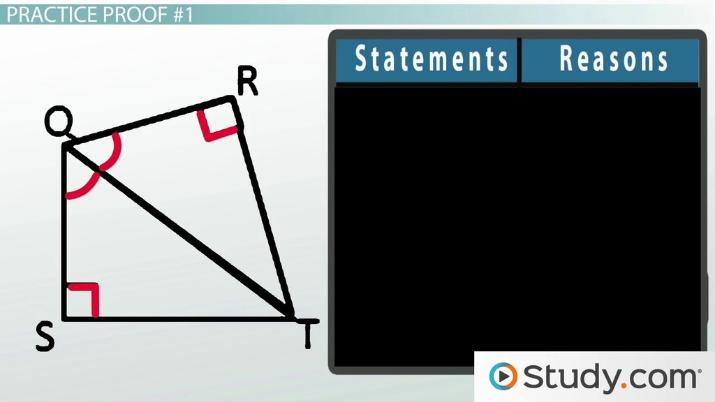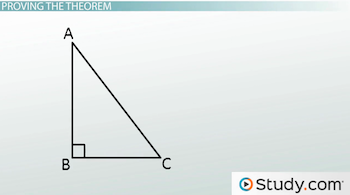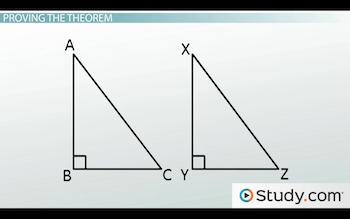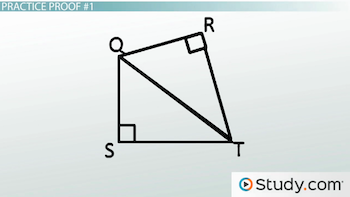# The HA (Hypotenuse Angle) Theorem: Proof, Explanation, & Examples

Lesson Transcript
Instructor: Jeff Calareso

Jeff teaches high school English, math and other subjects. He has a master's degree in writing and literature.

The hypotenuse angle or HA theorem states that if a hypotenuse and acute angle of a right triangle is identical to the angles of another right triangle, the two are congruent. Use a series of examples to learn how to prove, explain, and practice the HA theorem. Updated: 07/08/2022

## The HA Theorem

If you're a triangle, finding out that you're congruent to another triangle is a big deal. Imagine finding out one day that you have a twin that you didn't know about. How amazing would that be? It's like having a spare 'you' suddenly enter your life.

In geometry, we try to find triangle twins in any way we can. There are all kinds of methods, like side-side-side, angle-side-angle, side-angle-side and more. In the real world, it doesn't work that way. You can't just compare legs with a stranger to test for congruency.

With two right triangles, we already know that they have something in common - those right angles. So, it's like they're at least cousins. And we can prove they're congruent with the hypotenuse angle theorem.

The hypotenuse angle theorem, also known as the HA theorem, states that 'if the hypotenuse and an acute angle of one right triangle are congruent to the hypotenuse and an acute angle of another right triangle, then the two triangles are congruent.'An error occurred trying to load this video.

Try refreshing the page, or contact customer support.

Coming up next: The HL (Hypotenuse Leg) Theorem: Definition, Proof, & Examples

### You're on a roll. Keep up the good work!

Replay
Your next lesson will play in 10 seconds
• 0:05 The HA Theorem
• 1:04 Proving the Theorem
• 2:24 Practice Proof #1
• 3:53 Practice Proof #2
• 5:10 Lesson Summary
Save Save

Want to watch this again later?

Timeline
Autoplay
Autoplay
Speed Speed

## Proving the Theorem

How can we verify congruency with just a hypotenuse and an acute angle? It's like saying two people are twins because they have the same height and hair color. Let's look at a couple of triangles.

Here's triangle ABC:Now along comes triangle XYZ:Could they be twins? They're both right triangles. Angles B and Y are each 90 degrees. We're told that AC is congruent to XZ. So, that's one hypotenuse that's congruent to the other. And we're told that angle A is congruent to angle X. That's good, but it's not like a DNA test.

Or is it? In triangle ABC, what's the sum of the interior angles? 180. What about with triangle XYZ? It's also 180. So, if two angles are congruent, like A and X, and another two angles are congruent, like B and Y, then the other angles, C and Z, must also be congruent.

So, now we have angle A, side AC and angle C congruent to angle X, side XZ and angle Z. And that's angle-side-angle, or ASA. That means that the HA theorem is really just a simplification or variation of the ASA postulate that works with right triangles.

## Practice Proof #1

Let's try to find some twins with some proofs. You know, you're not twins without proof. Here are two triangles:They're very close. Together, they look kinda like a kite, don't they? Maybe they like to fly kites together. But are they just really good friends, or are they twins?

We're given that angles R and S are right angles. And we're also given that angle SQT is congruent to angle RQT. Let's say we want to determine if RT is congruent to ST.

Let's start our proof by collecting DNA samples from each triangle. Wait, what? Two-dimensional polygons don't have DNA? Oh. Then I guess we'll need to do an ordinary proof. Okay, first, we know that angles R and S are right angles. We're given that. That means that triangles QST and QRT are right triangles. That's the definition of a right triangle.

To unlock this lesson you must be a Study.com Member.

### Register to view this lesson

Are you a student or a teacher?